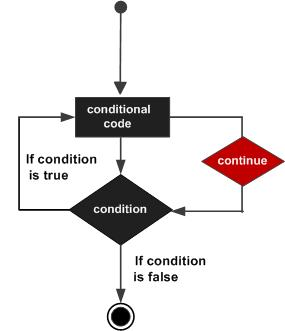# MATLAB - continue Statement

The continue statement is used for passing control to next iteration of for or while loop.

The continue statement in MATLAB works somewhat like the break statement. Instead of forcing termination, however, 'continue' forces the next iteration of the loop to take place, skipping any code in between.

## Flow Diagram## Example

Create a script file and type the following code −

```a = 9;
%while loop execution
while a < 20
a = a + 1;
if a == 15
% skip the iteration
continue;
end
fprintf('value of a: %d\n', a);
end
```

When you run the file, it displays the following result −

```value of a: 10
value of a: 11
value of a: 12
value of a: 13
value of a: 14
value of a: 16
value of a: 17
value of a: 18
value of a: 19
value of a: 20
```
matlab_loops.htm